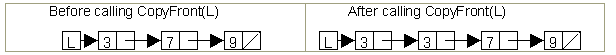Use this node definition on the questions that follow.

```	struct node
{
int info;
node * next;
node (int D, node *N)
: info (D),
next (N)
{ } 		// all fields initialized in initializer list
} ;			// notice semi-colon
```
1. Draw a diagram and display the output:
```	node *r = new node(9, NULL);
node *s = new node(7, r);
node *p = new node(3, s);
cout << p->info + s->info + r->info << endl;
```
2. Complete this swap function:
```	void swap (node*a, node*b)
// pre:	 a and b are initialized and not NULL
// post: the data stored in the info fields of a and b have been exchanged
{
```
3. Consider function `CopyFront` outlined below:
```	void CopyFront (node *L)
// pre:	 L is not NULL
// post: the first node in list L has been copied and inserted after the first node
{
< more code >
}
```
For example:Which of the following code segments could be used to replace `< more code> ` so that `CopyFront` works as intended?
```	A.  L = new node (L->info, NULL);
B.  L = new node (L->info, L);
C.  L = new node (L->info, L->next);
D.  L->next = new node (L->info, L);
E.  L->next = new node (L->info, L->next);
```

Continue to:  Unit 6  / Prev  / Next